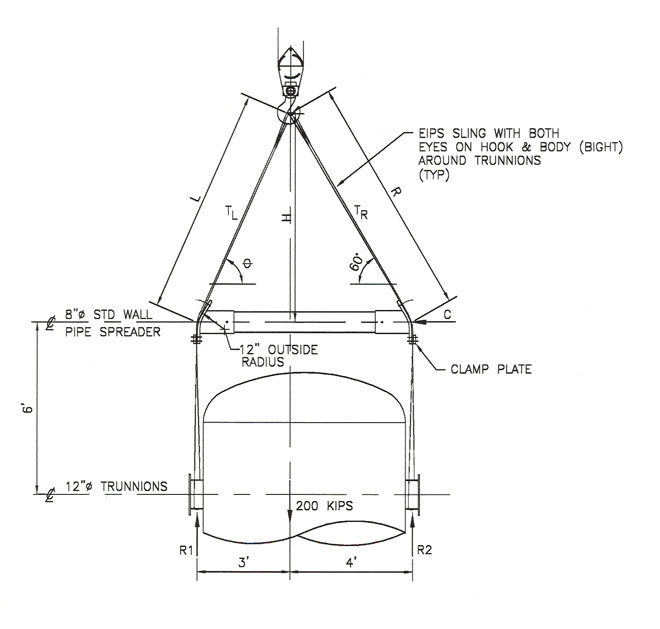Maximum Reach Quiz No. 22FIND: 1. Unknown angle, forces and lengths where C = the compressive force in the pipe, TL and TR = the tension in the inclined portions of the slings above the pipe spreader bar. 2. Determine the required diameter of the doubled slings using EIPS wire rope (for both the left and right sides.) 3. Find the total length of the doubled slings (both left and right) that will ensure that the load will hang level. 4. Does the force C put a bending moment in the pipe spreader bar as shown? 5. Is the force C the same value at both ends of the pipe spreader bar? 6. What is the value of the critical force pushing down on the pipe spreader bar? 7. What keeps the pipe spreader bar from slipping down the slings when it is under load? 8. List three ways that the pipe spreader bar is adjustable. (a.)___________ (b.)___________ (c.)___________ Maximum Reach Enterprises